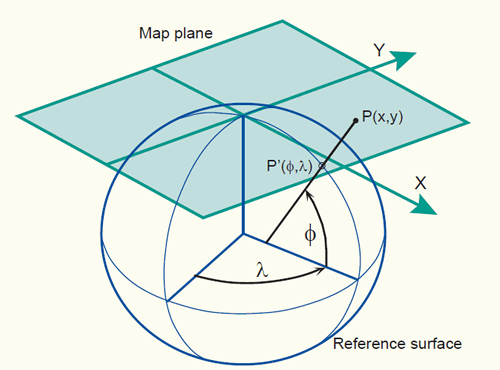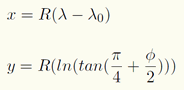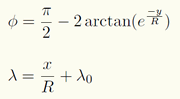## Mapping the sphere

It is not possible to map a portion of the sphere into the plane without introducing some distortion. There is a lot of evidence for this. For one thing you can do a simple experiment. Cut a grapefruit in half and eat one of the halves. Now try to flatten the remaining peel without the peel tearing. If that is not convincing enough, there are mathematical proofs. One of the nicest uses the formulas for the sum of the angles of a triangle on the sphere and in the plane. The fact that these are different shows that it is not possible to find a map from the sphere to the plane which sends great circles to lines and preserves the angles between them. The question then arises as to what is possible. That is the subject of these pages.

We will present a variety of maps and discuss the advantages and disadvantages of each. The easiest such maps are the central projections. Two are presented, the gnomonic projection and the stereographic projection. Although many people think so, the most important map in navigation, the Mercator projection, is not a central projection, and it will be discussed next. Finally we will talk briefly about a map from the sphere to the plane which preserves area, a fact which was observed already by Archimedes and used by him to discover the area of a sphere. All of these maps are currently used in mapping the earth. The reader should consult an atlas, such as those published by Rand Macnally, or the London Times. On each of the charts in such an atlas the name of the projection used will be indicated. The variety of projections used may be surprising.

There is a quantitative way of measuring distortion, and how it changes from place to place on the sphere. The distortion ellipse provides a way of graphically displaying this information. We will compute and display the distortion ellipse for each of the maps we discuss.

file:///G:/CARTOGRAFIA/cartstereographic.pdf

Introduction

Maps are one of the world’s oldest types of document. For quite some time it was thought that our planet was flat, and during those days, a map simply was a miniature representation of a part of the world. Now that we know that the Earth’s surface is curved in a specific way, we know that a map is in fact a flattened representation of some part of the planet. The field of map projections concerns itself with the ways of translating the curved surface of the Earth into a flat map.

What is a map projection?

map projection is a mathematically described technique of how to represent the Earth’s curved surface on a flat map. To represent parts of the surface of the Earth on a flat paper map or on a computer screen, the curved horizontal reference surface must be mapped onto the 2D mapping plane. The reference surface for large-scale mapping is usually an oblate ellipsoid, and for small-scale mapping, a sphere. Mapping onto a 2D mapping plane means transforming each point on the reference surface with geographic coordinates (f,l) to a set of Cartesian coordinates (x,y) representing positions on the map plane (figure below).Example of a map projection where the reference surface with geographic coordinates (f,lis projected onto the 2D mapping plane with 2D Cartesian coordinates (x, y).

The actual mapping cannot usually be visualized as a true geometric projection, directly onto the mapping plane as illustrated in the figure above. This is mostly achieved through mapping equations. A forward mapping equation transforms the geographic coordinates (f,l) of a point on the curved reference surface to a set of planar Cartesian coordinates (x,y), representing the position of the same point on the map plane:

(x, y) = (f, l)

The corresponding inverse mapping equation transforms mathematically the planar Cartesian coordinates (x,y) of a point on the map plane to a set of geographic coordinates (f,l) on the curved reference surface:

(f, l) = f (x, y)

Following are two examples of mapping equations for the sphere (equations for the ellipsoid are generally more complex).

1. The first example are the mapping equations used for the Mercator projection:

The forward mapping equation is:The inverse mapping equation is:where R is the radius of the spherical reference surface; f and l are given in radians; lo is the central meridian of the projection; e = 2.7182818, the base of the natural logarithms, not the eccentricity.

Suppose a point, located at 60oN and 130oW, is projected on a map that uses the Mercator projection (where the reference surface is a sphere with a radius of 6371000 m. and the central meridian (lo) is 0o, equal to the Greenwich meridian). Using the forward mapping equation of the Mercator projection, the values found for the Cartesian coordinates are for x = -14,455,340m and for = 8,390,339m.

https://kartoweb.itc.nl/geometrics/Map%20projections/body.htm